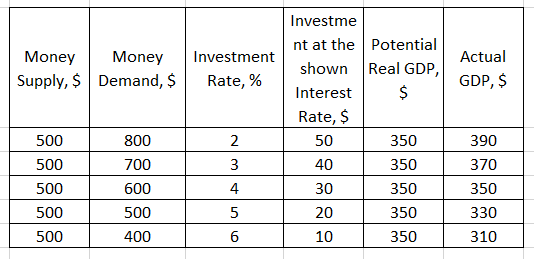# Refer to the table for Moola to answer the following questions

Refer to the table for Moola to answer the following questions.a) What is the equilibrium interest rate in Moola?
b) What is the level of investment at the equilibrium interest rate?
c) Is there either a recessionary output gap (negative GDP gap) or an inflationary output gap (positive GDP gap) at the equilibrium interest rate and, if either, what is the amount?
d) Given money demand, by how much would the Moola central bank need to change the money supply to close the output gap?
e) What is the expenditure multiplier in Moola?